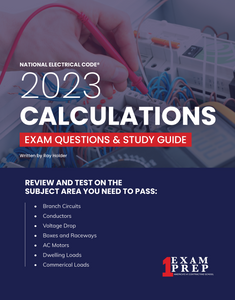# 2023 Practical Calculations for Electricians [Book]

• \$49.95
• Save \$5.55
Shipping calculated at checkout.

### 2023 Practical Calculations for Electricians (Book)

This self-study exam prep book is based on the 2023 NEC® with ten practice calculations exams consisting of 25 questions each and a final exam of 100 questions. This calculations book covers most topics that are included on all Journeyman and Master Electricians exams such as:

• Conductor sizing and protection
• Motors
• Transformers
• Voltage drop
• Box and conduit sizing
• Over-current protection
• Residential and commercial load calculations

The text contains the most widely used electrical calculations and formulas the reader needs to pass the journeyman and master electrical competency exam.

• Ten 25 Question Practice Exams with Answers
• 100 Question Final Exam with Answers and Analysis
• Helpful Tips to Pass the Test

This comprehensive electrical calculations textbook is based on the 2023 NEC® and contains complete coverage of core concepts of electrical calculations needed by every electrician. This book is arranged with topic-by-topic organization and step-by-step calculation procedures giving the electrician insight and understanding to solving mathematical problems. The book will familiarize you with formulas and calculations for branch circuits, AC motors, voltage drop, power factor, conductors, boxes & raceways, appliances, dwellings, commercial occupancies, and many more topics.

ISBN: 979-8377459255